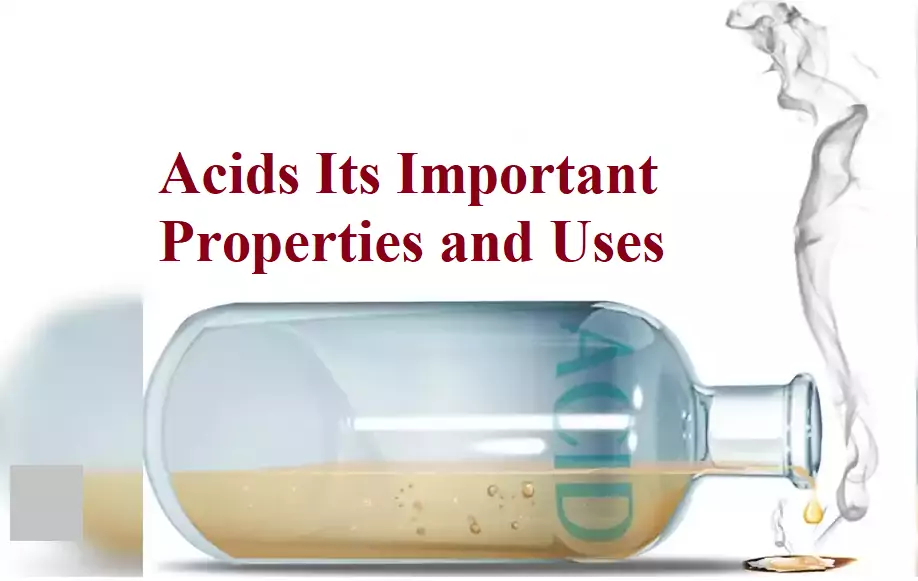# Acids Its Important Properties and UsesAcids Its Important Properties and Uses

ACIDS: Acid is a substance which furnishes H+ ions or H3O+ ions when dissolved in water. Acids have one or more replaceable hydrogen atoms. The word acid is derived from the Latin name ‘Acidus’ which means Sour Taste. Substances with ‘sour taste’ are acids. Lemon juice, vinegar and grape juice have a sour taste, so they are acidic.
Substances which turn blue litmus solution red are called acids.

CLASSIFICATION OF ACIDS:
1. Based on Source: Acids are of two types Mineral acids (Inorganic acid), Organic acid.
(a) Acids which are obtained from minerals and rocks are called Mineral acids. For e.g., H2SO(Sulphuric acid), HNO(Nitric acid) and HCl (Hydrochloric acid).
(b) Acids which are extracted from plants and animals are called Organic acids. For e.g. Citric acid (C6H8O7), Ascorbic acid (C6H8O6), Tartaric acid (C4H6O6), Lactic acid (C3H6O3), Acetic acid (C2H4O2).

2. Based on ionisation: Acids are classified into two types based on ionisation.
(a) Strong acids: Completely dissociate into its ions in aqueous solutions. Example: Nitric acid (HNO3), Sulphuric acid (H2SO4), Hydrochloric acid (HCl).
(b) Weak acids: Which do not completely dissociate into its ions in aqueous solutions. For example Carbonic acid (H2CO3), acetic acid (CH3COOH).

3. Based on concentration: Depending on the percentage or amount of acid dissolved in water acids are classified into dilute acid and concentrated acid.
(a) Dilute acids: Have a low concentration of acids in aqueous solutions.
(b) Concentrated acids: Have a high concentration of acids in aqueous solutions.

4. Based on their number of Hydrogen ion acids are:
(a) Monoprotic acid: Such type of acid produces one mole of H+ ions per mole of acid, e.g., HCl, HNO3.
(b) Diprotic acid: They can produce two moles of H+ ions per mole of acid, e.g., H2SO4.
(c) Triprotic acid: They produce three moles of H+ ions per mole of acid, e.g., H3PO4.
(d) Polyprotic: They can produce more than three H+ ions per mole of acid.

CHEMICAL PROPERTIES OF ACIDS:
(a) The reaction of Acids With Metal:
Acids react with active metals to give Salt and Hydrogen gas.
Metal + Acid → Salt + Hydrogen
For Example:
Hydrogen gas and Zinc Chloride formed when Zinc Metal reacts with Sulphuric acid.
Zn    +    H2SO4    →    ZnSO4    +     H2
Hydrogen gas and Sodium Chloride formed when Sodium Metal reacts with Hydrochloric acid.
2 Na    +    2 HCl    →   2 NaCl   +    H2

Acids Its Important Properties and Uses

(b) Reaction of Acids with Metal Carbonate:
Acids react with metal carbonates to form salt and water with the evolution of carbon dioxide gas.
Metal Carbonate + Acid → Salt + Carbon dioxide + Water
For Example:
Sulphuric acid gives calcium sulphate, carbon dioxide gas, calcium sulphate and water when it reacts with calcium carbonate.
CaCO3 + H2SO4 → CaSO4 + CO2 + H2O
Hydrochloric acid reacts with sodium carbonate to form sodium chloride and water with the release of carbon dioxide gas.
Na2CO3(s)    +   2 HCl(aq)   →    2NaCl(aq)   +   CO2(g)  + H2O(l)
Hydrochloric acid gives carbon dioxide, magnesium chloride and water when it reacts with magnesium carbonate.
MgCO3 + 2HCl → MgCl2 + CO2 + H2O
Hydrochloric acid gives carbon dioxide gas, calcium chloride and water when it reacts with calcium carbonate.
CaCO3 + 2HCl → CaCl2 + CO2 + H2O

(c) Reaction of Acid With Hydrogen Carbonates (Bicarbonates):
Acids give carbon dioxide gas, respective salt and water when they react with metal hydrogen carbonate.
Acid + Metal hydrogen carbonate → Salt + Carbon dioxide + Water
Sodium bicarbonate also reacts with hydrochloric acid to form sodium chloride and water with the release of carbon dioxide gas.
NaHCO3(s)       +     HCl (aq) →    NaCl (aq)   +   CO2(g) + H2O(l)
Sulphuric acid gives sodium sulphate, carbon dioxide gas and water when it reacts with sodium bicarbonate.
2 NaHCO3 + H2SO4 → Na2SO4 + 2CO2 + 2H2O

Acids Its Important Properties and Uses

USES of ACIDS:
(i) Sulphuric acid (King of chemicals) is used in the car battery and in the preparation of many other compounds
(ii) Nitric acid is used in the production of ammonium nitrate which is used as fertilizer in agriculture.
(iii) Hydrochloric acid is used as a cleansing agent in the toilet.
(iv) Tartaric acid is a constituent of baking powder.
(v) Salt of benzoic acid (sodium benzoate) is used in food preservation.
(vi) Carbonic acid is used in aerated drinks.

Acids Its Important Properties and Uses